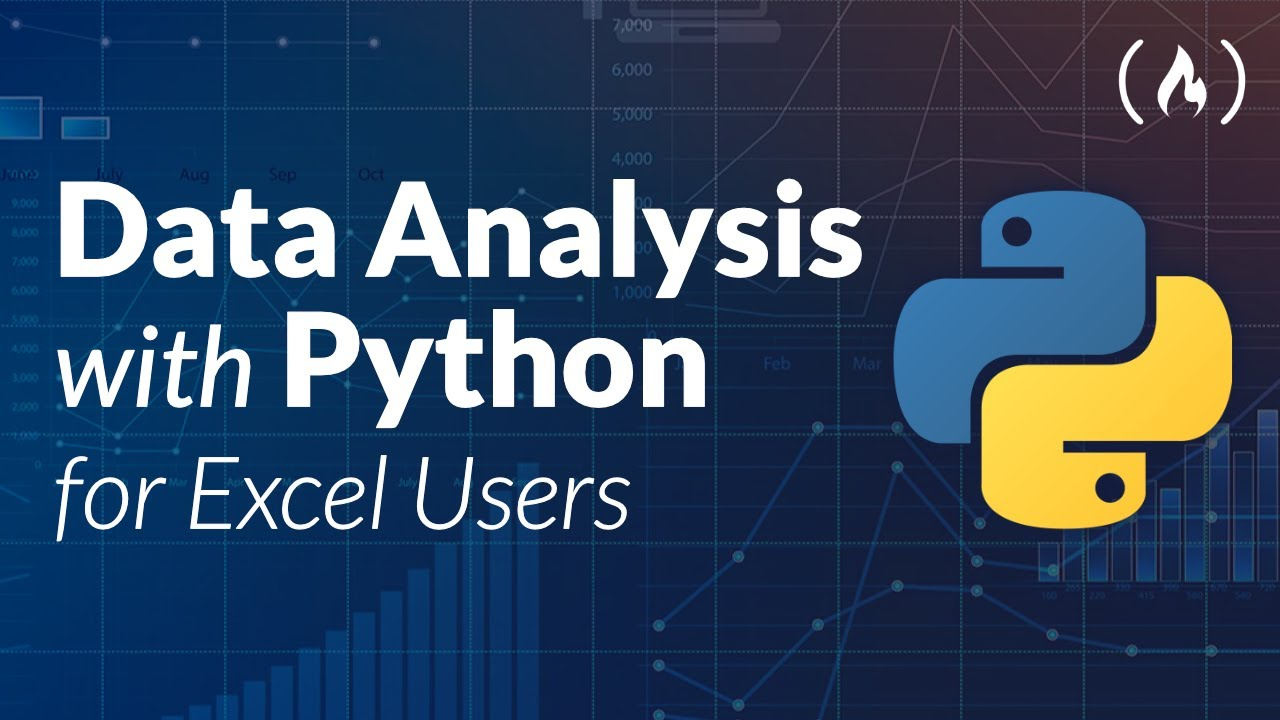# Data Analysis with Python for Excel Users - Full CourseLearn how to use Python and Pandas for data analysis. This course will be especially helpful if you have experience with Excel,

Learn how to use Python and Pandas for data analysis. This course will be especially helpful if you have experience with Excel, but that is not required. Learn how to create pivot tables, work with data, and make visualizations using Python, Pandas, and Jupyter Notebook.

⭐️ Course Contents ⭐️
⌨️ (0:00:00) Intro
⌨️ (0:01:48) Install Python and Jupyter Notebook with Anaconda
⌨️ (0:03:53) Jupyter Notebook Interface
⌨️ (0:13:56) Cell Types and Cell Mode
⌨️ (0:21:34) Jupyter Notebook Shortcuts
⌨️ (0:26:39) Module 1 - Hello World
⌨️ (0:30:30) Data Type
⌨️ (0:39:10) Variables
⌨️ (0:46:53) Lists
⌨️ (1:11:14) Dictionaries
⌨️ (1:21:50) IF Statement
⌨️ (1:28:04) FOR Loop
⌨️ (1:33:49) Functions
⌨️ (1:40:59) Modules
⌨️ (1:44:41) Module 2 -Introduction to Pandas
⌨️ (1:51:08) How to create a dataframe
⌨️ (2:07:34) How to show a dataframe
⌨️ (2:14:24) Basic Attributes, Functions and Methods
⌨️ (2:26:17) Selecting One Column from a Dataframe
⌨️ (2:32:13) Selecting Two or More Columns from a Dataframe
⌨️ (2:37:50) Add New Column to a Dataframe (Simple Assignment)
⌨️ (2:47:51) Operations in dataframes
⌨️ (2:56:04) The value_counts() method
⌨️ (3:00:16) Sort a DataFrame with the sort_values() method
⌨️ (3:09:56) Module 3: Introduction to Pivot Tables in Pandas
⌨️ (3:14:42) The pivot() method
⌨️ (3:20:49) The pivot_table() method
⌨️ (3:29:00) Data Visualization with Pandas (New Dataset + Pivot Table)
⌨️ (3:38:38) Lineplot
⌨️ (3:43:05) Barplot
⌨️ (3:50:52) Piechart
⌨️ (3:54:36) Save Plot and Export Pivot Table